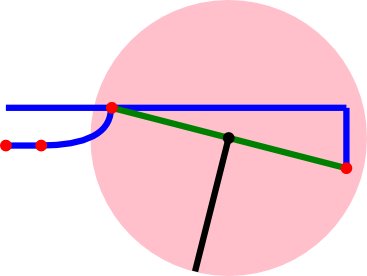# Simultaneous solving of equations â€“ who knew it would come to this?

Oh yeah.Â  I had a shoulder line with a slope.Â  Needed to make aÂ  dart perpendicular to shoulder line at the midpoint. Aha!Â  Get slope of shoulder line, negate & invert to get perpendicular slope.Â  Use midpoint as center point of circle. Solve line and circle equations simultaneously.Â ÂMy issues were that python doesn’t like ^ for exponents, likes ** instead.Â  Use as many parentheses as you can possibly stand, didn’t get the right results withÂ  r / (1+m**2)**(1/2) .Â Â  This was changed to (r/((1+m**2)**(.5))) and it worked perfectly.Â  And did you notice my Quadratic Bezier Curve?